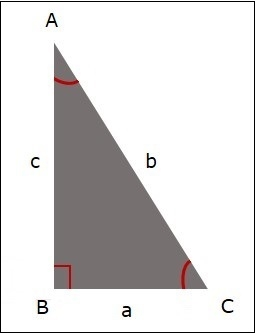# Java Program to find all angles of a triangle

To find the angles of a triangle we can use sin/cosine rules according to cosine rule −

cos A = (b^2 + c^2 - a^2)/2bcwhere A, B , C are the vertices and a, b, c are the sides of the given triangle then, angles of the triangle when the sides a, b, c given are −

angleAtA = acos((b^2 + c^2 - a^2)/(2bc))
angleAtB = acos((a^2 + c^2 - b^2)/(2ac))
angleAtC = acos((a^2 + b^2 - c^2)/(2ab))